How Cheenta works to ensure student success?
Explore the Back-Story

# Indian National Math Olympiad, INMO 2018 Problems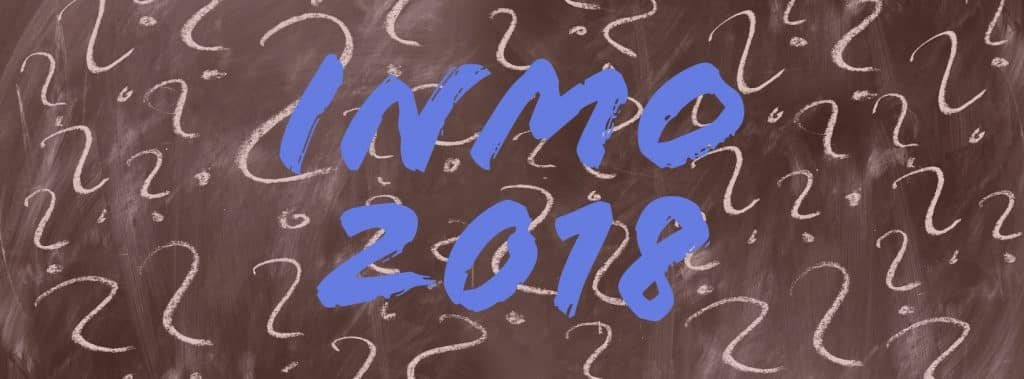This post contains problems from Indian National Mathematics Olympiad, INMO 2018. Try them and share your solution in the comments.

INMO 2018, Problem 1

Let ABC be a non-equilateral triangle with integer sides. Let D and E be respectively the mid-points BC and C A, let G be the centroid of triangle ABC. Suppose D, C, E, G are concyclic. Find the least possible perimeter of triangle ABC.

INMO 2018, Problem 2

For any natural number n, consider arectangular board made up of n unit squares. This is covered by three types of tiles;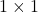red tile,green tile and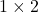blue domino. (For example, we can have 5 types of tiling when n = 2; red-red; red-green; green-red; green-green; and  blue.) Letdenote the number of ways of covering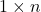rectangular board by these three types of tiles. Prove thatdivides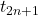.

INMO 2018, Problem 3

Let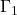and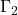be two circles with respective centresand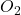intersecting in two distinct points A and B such that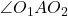is an obtuse angle. Let the circumcircle of triangle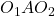intersectrespectively in points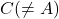and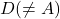. Let the line C B intersectin E; let the line D B intersectin F . Prove that the points C, D, E, F are concyclic.

INMO 2018, Problem 4

Find all polynomials with real coefficients P(x) such that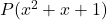divides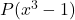.

INMO 2018, Problem 5

There are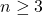girls in a class sitting around a circular table, each having some apples with her, Every time the teacher notices a girl having more apples than both of her neighnors combined, the teacher takes away one apple from that girl and gives one apple each to her neighbors. Prove that this process stops after a finite numberof steps. (Assume that the teacher has an abundant supply of apples.)

INMO 2018, Problem 6

Let N denote the set of all natural numbers and let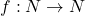be a function such that
(a)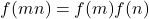for all m,n in N ;
(b) m+n divides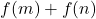for all m, n in N
Prove that there exists an odd natural number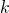such that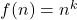for all n in N.

This post contains problems from Indian National Mathematics Olympiad, INMO 2018. Try them and share your solution in the comments.

INMO 2018, Problem 1

Let ABC be a non-equilateral triangle with integer sides. Let D and E be respectively the mid-points BC and C A, let G be the centroid of triangle ABC. Suppose D, C, E, G are concyclic. Find the least possible perimeter of triangle ABC.

INMO 2018, Problem 2

For any natural number n, consider arectangular board made up of n unit squares. This is covered by three types of tiles;red tile,green tile andblue domino. (For example, we can have 5 types of tiling when n = 2; red-red; red-green; green-red; green-green; and  blue.) Letdenote the number of ways of coveringrectangular board by these three types of tiles. Prove thatdivides.

INMO 2018, Problem 3

Letandbe two circles with respective centresandintersecting in two distinct points A and B such thatis an obtuse angle. Let the circumcircle of triangleintersectrespectively in pointsand. Let the line C B intersectin E; let the line D B intersectin F . Prove that the points C, D, E, F are concyclic.

INMO 2018, Problem 4

Find all polynomials with real coefficients P(x) such thatdivides.

INMO 2018, Problem 5

There aregirls in a class sitting around a circular table, each having some apples with her, Every time the teacher notices a girl having more apples than both of her neighnors combined, the teacher takes away one apple from that girl and gives one apple each to her neighbors. Prove that this process stops after a finite numberof steps. (Assume that the teacher has an abundant supply of apples.)

INMO 2018, Problem 6

Let N denote the set of all natural numbers and letbe a function such that
(a)for all m,n in N ;
(b) m+n dividesfor all m, n in N
Prove that there exists an odd natural numbersuch thatfor all n in N.

This site uses Akismet to reduce spam. Learn how your comment data is processed.

### Knowledge Partner